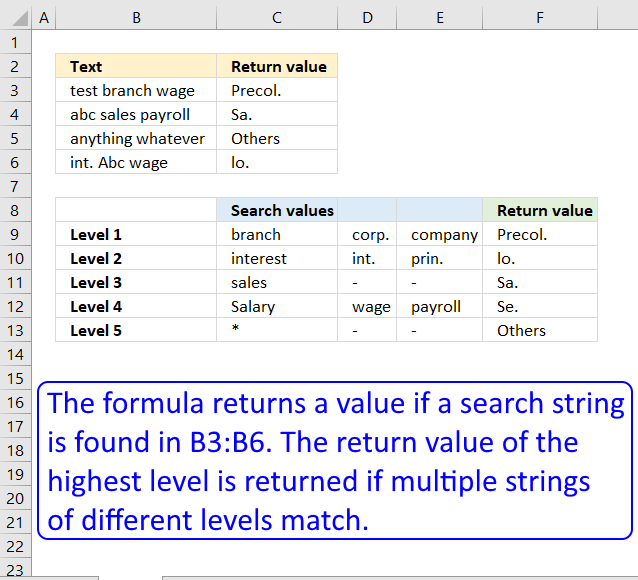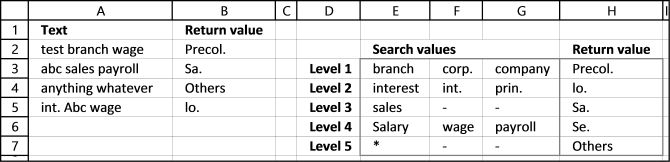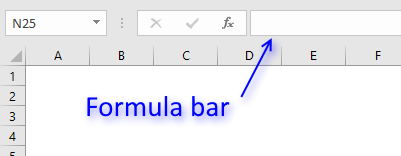Author: Oscar Cronquist Article last updated on February 03, 2023This article demonstrates a formula that searches a cell (partial match) based on values in a table and returns a value based on its position. The value with the highest level is returned if two matches are found.

For example, cell B3 in the above image contains the value "test branch wage", it matches both "branch" in cell C9 and "wage" in cell D12 however, only the corresponding value with the highest level is returned. "Level 1" is higher than "Level 2".

Value "branch" is on a higher level than the value "wage" and the corresponding value to "branch" is "Precol.", value "Precol." is returned to cell C3.

Hello Mr Oscar
I have the matter to create a mega formula to categorize my list. For short example:A1: Cash in deposit (Branch A t/t)
A2: Borrowed from Corp. A
A3: Interest payment
A4: Int.penalty pmt
A5: Prin. Pmt
A6: Salary Pmt on April
A8: Branch C t/t
A9: Transferred from Company AA
A10: Mortgages to DD ltd
A11: Sal. Pmt on Mayand
at B1 cell, I create a formula as follows:=IF(COUNT(SEARCH({"branch","corp.", "company"},A1))>0,"Precol.", IF(COUNT(SEARCH({"interest","int.", "prin."},A1))>0,"lo.",IF(COUNT(SEARCH("sales", A1))>0,"Sa.",IF(COUNT(SEARCH({"sal.","Salary", "wage","payroll"},A1))>0,"Se.","Others"))))But, my formula is too long and too many parentheses. I want to shorten this formula or replace it with another. But how? Could you please solve my question? Thank you very much.HungIt looks like your formula has different levels with search words. The word found with the lowest level should be returned, leave the remaining.

Example 1, cell A2 contains "test branch wage". "branch" is found on level 1 (cell E3) and "wage" on level 4 (cell F6). However "branch" is on the lowest level of the two so Precol. (cell H3) is returned in cell B2.

Example 2, cell A3 contains "abc sales payroll". "sales" is a search string found on level 3 and "payroll" is on level 4. Level 3 is the lowest level so "Sa." (cell H5) is returned in cell B3.

Example 3, cell A4 contains"anything whatever" and no search value is found except the asterisk (*) on level 5.  Text string "Others" (cell H7) is returned in cell B4.=INDEX(\$F\$9:\$F\$13, MIN(IF(ISNUMBER(SEARCH(\$C\$9:\$E\$13, B3)), MATCH(ROW(\$C\$9:\$E\$13), ROW(\$C\$9:\$E\$13)), "")))

### How to enter an array formula

1. Select cell B2.
2. Type the formula above in the formula bar.3. Press and hold CTRL + SHIFT key.
4. Press Enter.

If you did the above steps correctly excel automatically adds a beginning and ending curly bracket {array_formula} to the formula. Don't enter these characters yourself.

### Explaining array formula in cell B2

You can easily follow along as I explain this array formula. Select cell B2 and go to "Formulas" on the ribbon, press with left mouse button on "Evaluate Formula" button. Press with mouse on "Evaluate" to go to next step.

#### Step 1 - Search for multiple text strings simultaneously

The SEARCH function returns the number of the character at which a specific character or text string is found. However, we are only interested if the string is found or not, this function is exactly what we need.

We are doing something strange with the SEARCH function below, we are not only using one string but multiple strings at once. That is why you see a cell range in the first argument.

SEARCH(find_text,within_text, [start_num])

SEARCH(\$E\$3:\$G\$7,A2)

becomes

SEARCH({"branch","corp.","company";"interest","int.","prin.";"sales","-","-";"Salary","wage","payroll";"*","-","-"},"test branch wage")

and returns

{6, #VALUE!, #VALUE!; #VALUE!, #VALUE!, #VALUE!;#VALUE!, #VALUE!,#VALUE!; #VALUE!,13,#VALUE!; 1,#VALUE!, #VALUE!}

The SEARCH function returns an array with the same size as the cell range used in the first argument, see above.

\$E\$3:\$G\$7 is a cell range containing multiple columns and rows. An array with multiple columns and rows uses commas and semicolons as delimiting characters.

A comma is used as a delimiting character to separate values column by column and a semi colon is used to separate values row by row.

\$E\$3:\$G\$7 has three columns, E, F and G. The returning array above contains three values separated by two commas and then a semicolon, so the array has also three columns. The same with the number of rows in cell range \$E\$3:\$G\$7 and rows in array.

#### Step 2 - Look for numbers in array

A number indicates that the text string is found, an error #VALUE tells you that no search string is found. The ISNUMBER function converts numbers to TRUE and all other values including errors to FALSE.

ISNUMBER(value)

ISNUMBER(SEARCH(\$E\$3:\$G\$7,A2))

becomes

ISNUMBER({6,#VALUE!,#VALUE!;#VALUE!, #VALUE!,#VALUE!;#VALUE!,#VALUE!,#VALUE!; #VALUE!,13,#VALUE!;1,#VALUE!,#VALUE!})

and returns {TRUE, FALSE, FALSE;FALSE, FALSE, FALSE;FALSE, FALSE, FALSE;FALSE, TRUE, FALSE;TRUE, FALSE, FALSE}

#### Step 3 - If text string is found return corresponding row number

The IF function returns one value if the logical test is TRUE and another value if the logical test is FALSE.

IF(logical_test, [value_if_true], [value_if_false])

IF(ISNUMBER(SEARCH(\$E\$3:\$G\$7, A2)), MATCH(ROW(\$E\$3:\$G\$7), ROW(\$E\$3:\$G\$7)),"")

becomes

IF({TRUE, FALSE, FALSE;FALSE, FALSE, FALSE;FALSE, FALSE, FALSE;FALSE, TRUE, FALSE;TRUE, FALSE, FALSE}, MATCH(ROW(\$E\$3:\$G\$7), ROW(\$E\$3:\$G\$7)), "")

becomes

IF({TRUE, FALSE, FALSE;FALSE, FALSE, FALSE;FALSE, FALSE, FALSE;FALSE, TRUE, FALSE;TRUE, FALSE, FALSE}, {1; 2; 3; 4; 5}, "")

and returns

{1,"","";"","","";"","","";"",4,"";5,"",""}

#### Step 4 - Extract smallest row number

The MIN function allows you to retrieve the smallest number in a cell range.

MIN(number1, [number2], ...)

MIN(IF(ISNUMBER(SEARCH(\$E\$3:\$G\$7, A2)), MATCH(ROW(\$E\$3:\$G\$7), ROW(\$E\$3:\$G\$7)), ""))

becomes

MIN({1,"","";"","","";"","","";"",4,"";5,"",""})

and returns 1.

#### Step 5 - Return value using row number

The INDEX function returns a value from a cell range, you specify which value based on a row and column number.

INDEX(array,row_num,[column_num])

INDEX(\$H\$3:\$H\$7, MIN(IF(ISNUMBER(SEARCH(\$E\$3:\$G\$7, A2)), MATCH(ROW(\$E\$3:\$G\$7), ROW(\$E\$3:\$G\$7)), "")))

becomes

INDEX(\$H\$3:\$H\$7, 1)

becomes

INDEX({"Precol.";"lo.";"Sa.";"Se.";"Others"}, 1)

and returns Precol. in cell B2.